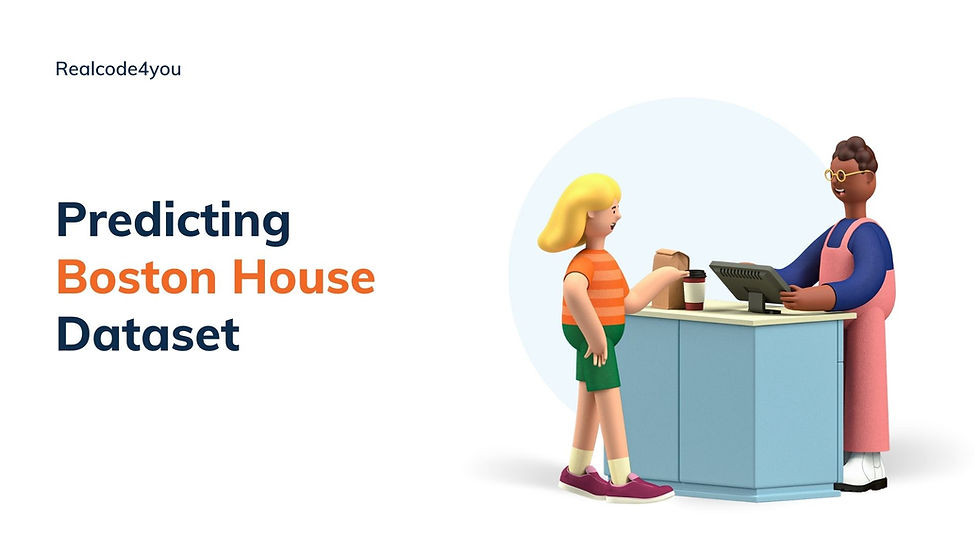top of page
Search

# Predicting Boston Housing Prices Using Python Machine LearningThe dataset for this project originates from the UCI Machine Learning Repository. The Boston housing data was collected in 1978 and each of the 506 entries represent aggregated data about 14 features for homes from various suburbs in Boston, Massachusetts. For the purposes of this project, the following preprocessing steps have been made to the dataset:

• 16 data points have an 'MEDV' value of 50.0. These data points likely contain missing or censored values and have been removed.

• 1 data point has an 'RM' value of 8.78. This data point can be considered an outlier and has been removed.

• The features 'RM', 'LSTAT', 'PTRATIO', and 'MEDV' are essential. The remaining non-relevant features have been excluded.

• The feature 'MEDV' has been multiplicatively scaled to account for 35 years of market inflation.

Run the code cell below to load the Boston housing dataset, along with a few of the necessary Python libraries required for this project. You will know the dataset loaded successfully if the size of the dataset is reported.

### Import Libraries

```import numpy as np
import pandas as pd
import visuals as vs # Supplementary code
from sklearn.model_selection import ShuffleSplit```

```# Pretty display for notebooks
%matplotlib inline

# Load the Boston housing dataset
prices = data['MEDV']
features = data.drop('MEDV', axis = 1)

# Success
print('Boston housing dataset has {0} data points with {1} variables each'.format(*data.shape))

```

Output

Boston housing dataset has 489 data points with 4 variables each

### Visualization

```# Using pyplot
import matplotlib.pyplot as plt
plt.figure(figsize=(20, 5))

# i: index
for i, col in enumerate(features.columns):

# 3 plots here hence 1, 3
plt.subplot(1, 3, i+1)
x = data[col]
y = prices
plt.plot(x, y, 'o')

# Create regression line
plt.plot(np.unique(x), np.poly1d(np.polyfit(x, y, 1))(np.unique(x)))
plt.title(col)
plt.xlabel(col)
plt.ylabel('prices')```

Output### Split Dataset

```# TODO: Import 'train_test_split'
from sklearn.model_selection import train_test_split

# TODO: Shuffle and split the data into training and testing subsets
X_train, X_test, y_train, y_test = train_test_split(features, prices, test_size=0.2, random_state=10)

# Success
print("Training and testing split was successful.")```

Output:

Training and testing split was successful.

### Fit Into the Model

Using Grid Search

```# TODO: Import 'make_scorer', 'DecisionTreeRegressor', and 'GridSearchCV'
from sklearn.metrics import make_scorer
from sklearn.tree import DecisionTreeRegressor
from sklearn.grid_search import GridSearchCV

def fit_model(X, y):
# Create cross-validation sets from the training data
cv_sets = ShuffleSplit(X.shape, n_iter = 10, test_size = 0.20, random_state = 0)

# TODO: Create a decision tree regressor object
regressor = DecisionTreeRegressor(random_state=0)

dt_range = range(1, 11)
params = dict(max_depth=dt_range)

# TODO: Create the grid search object
# You would realize we manually created each, including scoring_func using R^2
grid = GridSearchCV(regressor, params, cv=cv_sets, scoring=scoring_fnc)

# Fit the grid search object to the data to compute the optimal model
grid = grid.fit(X, y)

# Return the optimal model after fitting the data
return grid.best_estimator_

```

Using RandomizedSearchCV

```# Import RandomizedSearchCV
from sklearn.grid_search import RandomizedSearchCV

# Create new similar function
def fit_model_2(X, y):

# n_iter is the number of re-shuffling & splitting iterations.
cv_sets = ShuffleSplit(X.shape, n_iter = 10, test_size = 0.20, random_state = 0)

regressor = DecisionTreeRegressor(random_state=0)
dt_range = range(1, 11)
params = dict(max_depth=dt_range)
scoring_fnc = make_scorer(performance_metric)

# TODO: Create the grid search object
rand = RandomizedSearchCV(regressor, params, cv=cv_sets, scoring=scoring_fnc)

# Fit the grid search object to the data to compute the optimal model
rand = rand.fit(X, y)

# Return the optimal model after fitting the data
return rand.best_estimator_```

### Making Predictions

```# Fit the training data to the model using grid search
reg = fit_model(X_train, y_train)

# Produce the value for 'max_depth'
print "Parameter 'max_depth' is {} for the optimal model.".format(reg.get_params()['max_depth'])```

Output

Parameter 'max_depth' is 4 for the optimal model.